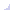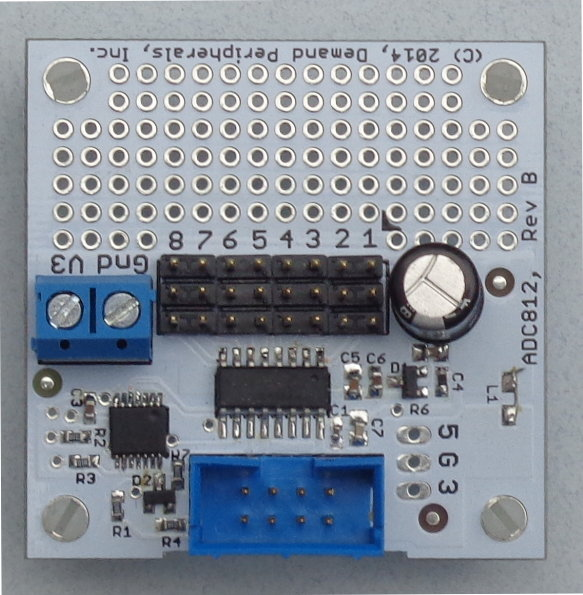Demand Peripherals     Robotics and Automation Made Easy

### ADC812: Eight Channel, 12-bit Analog-to-Digital Converter

The ADC812 peripheral performs a 12 bit ADC on eight inputs at a rate between 4 HZ and 100 Hz. Two inputs can be combined to form a differential input for the ADC. Differential inputs perform a 13 bit conversion.

Hardware:Resources:

The resources for the ADC812 let you specify the sample rate and whether or not an input is differential. The ADC samples are available at the 'samples' resource.

config : The sample period in milliseconds and an hex value to specify which inputs are differential. The sample period is given in decimal and must be between 10 and 256.

The differential inputs are given as an 8 bit hexadecimal number with a leading '0x'. If differential bit 0 is set then value 0 reports the signed 13-bit value of input 0 minus input 1. If bit 1 is set then value 1 reports the value of input 1 minus input0. This pattern is the same for the other differential pairs, 2/3. 4/5, and 6/7.

This is a read-write resource and works with dpset and dpget but not dpcat.

samples : eight space-separated 12-bit ADC readings as hex values. There is one line of output for each set of samples. Single-ended inputs give an unsigned 12 bit result and differential inputs give a signed 12 bit result (since the difference can be above or below the adjoining pin). You can use select() on this resource after giving the dpcat command.

Examples:

Set the sample rate to 50 milliseconds and eight single-ended inputs.

`   dpset adc812 config 50 0x00 `

`   dpcat adc812 samples `

If you have two ADC812 cards in your system you access the second one by slot number.
`   dpcat 3 samples `FPGA Defined Peripherals
User Interface
FPGA Configuration
FPGA Buttons & LEDs
Host Tx/Rx Interface
AVR Interface
Stereo Audio Amplifier
IR Recv/Xmit
6 Digit LCD
RC Decoder
Keyfob RF Decoder
Rotary Encoder Interface
Motion Control
Dual DC Motor Controller
Bipolar Stepper Controller
Unipolar Stepper Controller
Simple Input / Output
Octal Input/Output
32 Channel Binary Output
32 Channel Binary Input
Octal Serial Output
Sensors
Pololu QTR Interface
Octal SRF04 Interface
Instrumentation
Generic I2C
Generic SPI
Octal 8-Bit DAC